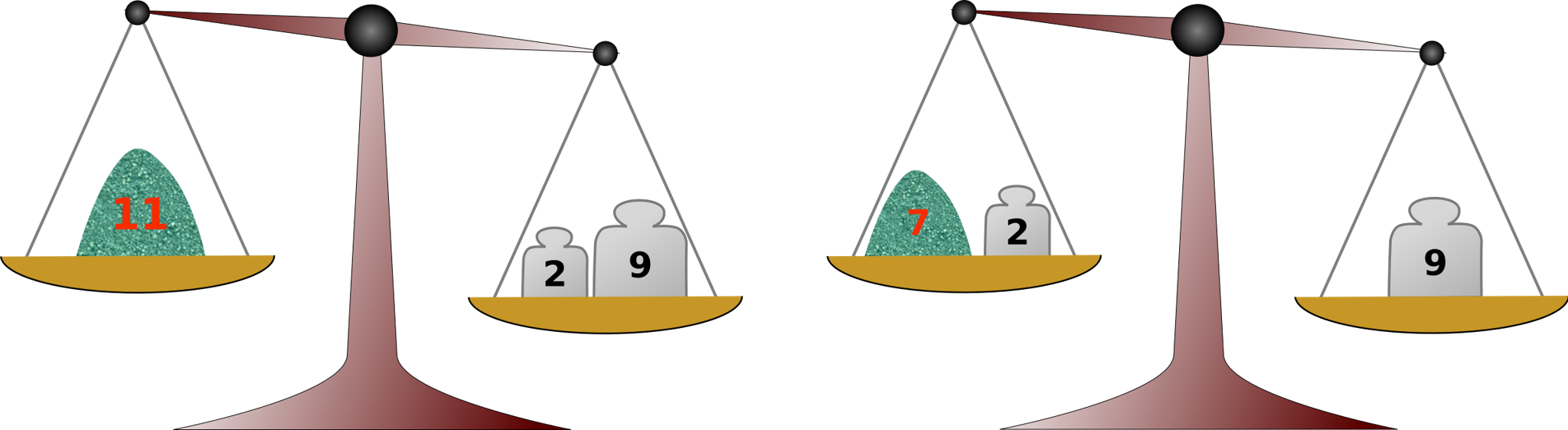시간 제한 메모리 제한 제출 정답 맞은 사람 정답 비율
2 초 512 MB 0 0 0 0.000%

문제

You, an experimental chemist, have a balance scale and a kit of weights for measuring weights of powder chemicals.

For work efficiency, a single use of the balance scale should be enough for measurement of each amount. You can use any number of weights at a time, placing them either on the balance plate opposite to the chemical or on the same plate with the chemical. For example, if you have two weights of 2 and 9 units, you can measure out not only 2 and 9 units of the chemical, but also 11 units by placing both on the plate opposite to the chemical (Fig. C-1 left), and 7 units by placing one of them on the plate with the chemical (Fig. C-1 right). These are the only amounts that can be measured out efficiently.Fig. C-1 Measuring 11 and 7 units of chemical

You have at hand a list of amounts of chemicals to measure today. The weight kit already at hand, however, may not be enough to efficiently measure all the amounts in the measurement list. If not, you can purchase one single new weight to supplement the kit, but, as heavier weights are more expensive, you'd like to do with the lightest possible.

Note that, although weights of arbitrary positive masses are in the market, none with negative masses can be found.

입력

The input consists of at most 100 datasets, each in the following format.

n m
a1 a2 ... an
w1 w2 ... wm

The first line of a dataset has n and m, the number of amounts in the measurement list and the number of weights in the weight kit at hand, respectively. They are integers separated by a space satisfying 1 ≤ n ≤ 100 and 1 ≤ m ≤ 10.

The next line has the n amounts in the measurement list, a1 through an, separated by spaces. Each of ai is an integer satisfying 1 ≤ ai ≤ 109, and aiaj holds for ij.

The third and final line of a dataset has the list of the masses of the m weights at hand, w1 through wm, separated by spaces. Each of wj is an integer, satisfying 1 ≤ wj ≤ 108. Two or more weights may have the same mass.

The end of the input is indicated by a line containing two zeros.

출력

For each dataset, output a single line containing an integer specified as follows.

• If all the amounts in the measurement list can be measured out without any additional weights, 0.
• If adding one more weight will make all the amounts in the measurement list measurable, the mass of the lightest among such weights. The weight added may be heavier than 108 units.
• If adding one more weight is never enough to measure out all the amounts in the measurement list, -1.

예제 입력 1

4 2
9 2 7 11
2 9
6 2
7 3 6 12 16 9
2 9
5 2
7 3 6 12 17
2 9
7 5
15 21 33 48 51 75 111
36 54 57 93 113
0 0

0
5
-1
5